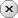### 用户注册### 用户登录### 发表随想# VsFlexgrid_DrawCell

2019-04-19 作者：Ex举报

## [vb]代码库

```Option Explicit
Private Type Rect
Left As Long
Top As Long
Right As Long
Bottom As Long
End Type
Private Declare Function CreatePen Lib "gdi32" (ByVal nPenStyle As Long, ByVal nWidth As Long, ByVal crColor As Long) As Long '创建画笔
Private Declare Function FrameRect Lib "user32" (ByVal hdc As Long, lpRect As Rect, ByVal hBrush As Long) As Long '画空心矩形
Private Declare Function FillRect Lib "user32" (ByVal hdc As Long, lpRect As Rect, ByVal hBrush As Long) As Long '画实心矩形
Private Declare Function DeleteObject Lib "gdi32" (ByVal hObject As Long) As Long '删除画笔
Private Const PS_SOLID As Long = 1
Private Sub Form_Load()
With List
Dim I As Integer
.Rows = 101
For I = 1 To 100
.TextMatrix(I, 1) = I
Next
.Cols = 3
.ExtendLastCol = True
.SelectionMode = 1 '选择整行
.Editable = flexEDKbdMouse
.AllowUserResizing = flexResizeBoth
.FocusRect = flexFocusNone '无虚框
.RowHeightMin = 300
.ColWidth(2) = 3000
.TextMatrix(0, 1) = "值"
.TextMatrix(0, 2) = "进度值"
.ColHidden(0) = True
.ColAlignment(1) = 4
.OwnerDraw = flexODOver
.Redraw = flexRDBuffered
End With
End Sub

Private Sub Form_Resize()
List.Move 0, 0, Me.ScaleWidth, Me.ScaleHeight
End Sub

Private Sub List_DrawCell(ByVal hdc As Long, ByVal Row As Long, ByVal Col As Long, ByVal Left As Long, ByVal Top As Long, ByVal Right As Long, ByVal Bottom As Long, Done As Boolean)
If List.TextMatrix(Row, 1) <> "" Then
Debug.Print Row
If Col = 2 And Row > 0 Then Percentage hdc, List.TextMatrix(Row, 1), Left, Top, Right, Bottom, RGB(0, 64, 128)
If Col = 2 And Row = List.Row Then Percentage hdc, List.TextMatrix(Row, 1), Left, Top, Right, Bottom, RGB(255, 255, 255)
End If

End Sub

Sub Percentage(ByVal hdc As Long, Value As Integer, ByVal Left As Long, ByVal Top As Long, ByVal Right As Long, ByVal Bottom As Long, ByVal crColor As Long)
Dim A As Long, B As Rect
A = CreatePen(PS_SOLID, 1, crColor) '创建画笔
B.Left = Left + 3
B.Top = Top + 3
B.Bottom = Bottom - 4
B.Right = Right - 4
FrameRect hdc, B, A

B.Left = Left + 5
B.Top = Top + 5
B.Bottom = Bottom - 6
B.Right = Right - 6
B.Right = B.Left + ((B.Right - B.Left) / 100 * IIf(Value < 0 Or Value > 100, 0, Value))

FillRect hdc, B, A
DeleteObject A
End Sub```

### 网友评论    (发表评论)### 评论须知：

• 1、评论每次加2分，每天上限为30；
• 2、请文明用语，共同创建干净的技术交流环境；
• 3、若被发现提交非法信息，评论将会被删除，并且给予扣分处理，严重者给予封号处理；
• 4、请勿发布广告信息或其他无关评论，否则将会删除评论并扣分，严重者给予封号处理。